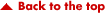Math Algebra Trig Pre-Calculus StatisticsTable Of Contents BASIC MATH AND ALGEBRAProvides a review of basic arithmetic and elementary algebra; includes fractions, decimals, percentages, exponents, radicals, logarithms; exercises in factoring polynomials, linear equations, ratio, proportion, variation, complex numbers and quadratic equations; presents brief introduction to plane figures, geometric construction, and trigonometry. Special Information: High school algebra or its equivalent is considered the minimum academic background for enrollment in this course. TRIGONOMETRYContains information on the following subjects: definitions, notations, and computations with logarithms; trigonometric ratios, analysis, applications, and aids to computations; trigonometric identities; and vectors and forces. This course is general in nature and is not directed toward any one specific specialty. Special Information: Successful completion of Mathematics, Part 1 or equivalent classroom courses in the fundamentals of algebra and trigonometry is strongly recommended prior to enrollment. PRE-CALCULUS AND INTRODUCTION TO PROBABILITYContains information on the following subjects: straight lines, conic sections, tangents, normals, slopes; introduction to differential and integral calculus; combinations and permutations; and introduction to probability. This course is general in nature and is not directed toward any specific specialty. Special Information: Successful completion of Mathematics, Part 1 and Mathematics, Volume 2-A or equivalent classroom courses in the fundamentals of algebra and trigonometry is strongly recommended prior to enrollment. INTRODUCTION TO STATISTICS, NUMBER SYSTEMS AND BOOLEAN ALGEBRAContains information on the following subjects: elements of digital computer mathematics--sequence and series, induction and the binomial theorem, statistics, number systems, sets and subsets, Boolean algebra, matrices, and determinants. Special Information: Successful completion of Mathematics, Part 1 or equivalent classroom courses prior to enrollment is strongly recommended.Copyright 2002 by WeMake CDs, Inc.PO Box 20158 Indianapolis, IN 46220